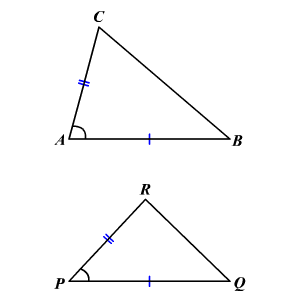# SAS Inequality

SAS Inequality

If two sides of a triangle are congruent to two sides of another triangle, and if the included angle of the first triangle is greater than the included angle of the second, then the third side of the first triangle is longer than the third side of the second.If $\stackrel{¯}{AB}\cong \stackrel{¯}{PQ},\stackrel{¯}{AC}\cong \stackrel{¯}{PR}$ , and if $m\angle A>m\angle P$ , then $BC>QR$ .